# [T01] Basic concepts## §1. The origins of probability theory

The notion of probability has been around for as long as people have gambled, and gambling has been around since ancient times. Everyone knows that some bets are riskier than others; betting that the next card drawn from a pack will be the queen of hearts is riskier than betting that it will be a heart. A successful gambler needs to be good at estimating the chance of winning a given bet. The notion of probability arose as a measure of chance; the higher the probability, the better the chance of winning.

Modern probability theory also arose from gambling. In the seventeenth century, mathematicians Blaise Pascal and Pierre de Fermat worked out the theory of probabilities as a response to a problem posed to Pascal by a gambler. These days, probability theory is far more than just a theory of gambling. It helps us with all kinds of risk assessment--in the insurance industry, in medical research, in engineering, and in virtually every other human endeavor.

Probability theory is the foundation of statistical reasoning, so if we are going to learn about statistical reasoning, we have to start with some probability theory. The first few sections cover the basics of probability theory, and each section ends with questions which enable you to check your understanding. The later sections contain examples of probabilistic reasoning, good and bad, including some of the most common mistakes people make when they use probabilities. If you already know how to calculate probabilities, you can skip straight to the examples.

## §2. Representing probabilities

A probability is represented by a number between 0 and 1. An event that is certain to happen is assigned a probability of 1. An event that is certain not to happen is assigned a probability of 0. To say that an event has some value in between means that it may or may not happen; the larger the probability, the more likely the event. To be more precise about what probability actually means is surprisingly difficult; click this button for a brief discussion of this topic:

Still, even if we can't say precisely what a probability is, we all know how to assign probabilities to simple events. For example, we all know that if we toss a coin, the probability of getting heads is 1/2. That's because there are two outcomes, heads and tails, and for a fair coin they have the same chance of occurring. Since the probabilities must add up to 1 (one or the other outcome is certain to happen), the probability of each outcome must be 1/2. Similarly, if you roll a six-sided die, there are six equally probable outcomes, so the probability of each outcome is 1/6.

As an abbreviation, it is customary to use a capital P to stand for probability. So, for example, we can abbreviate "The probability of getting heads when I toss this coin is 1/2" as follows:

P(H)=1/2

The symbol inside the parentheses stands for the outcome in question; I've used the letter H to stand for getting heads. Unless it's obvious, you need to state the meaning of the symbol you use to stand for the outcome.

## §3. Probabilities and odds

Odds are sometimes used instead of probability as a measure of chance. For example, suppose you are told that the odds of catching flu this year are 200:1 (read "two-hundred to one"). The sizes of the numbers on either side of the colon represent the relative chances of not catching flu (on the left) and catching flu (on the right). In other words, what you are told is that the chance of not catching flu is 200 times as great as the chance of catching flu.

Odds are usually presented in terms of whole numbers. So if you want to say that the chance of Lee losing the election is two and a half times as great as the chance of him winning, you would express this by saying that the odds of Lee winning are 5:2. The number on the left (the chance of him losing) is two and a half times bigger than the number on the right (the chance of him winning).

Note that odds of 10:1 are not the same as a probability of 1/10. If an event has a probability of 1/10, then the probability of the event not happening is 9/10. So the chance of the event not happening is nine times as great as the chance of the event happening; the odds are 9:1.

1. What are the odds that tossing a fair coin will produce heads?
2. What are the odds that rolling a fair die will produce a 6?
3. Suppose the odds of the horse Blaise winning the 8 o'clock race at Happy Valley are 25:1 (and suppose that this actually represents the chance that the horse will win). What is the probability that Blaise will win?
4. If the odds of Lee winning the election are 5:2, what is the probability of him winning?
5. If the odds of an event are x:y, what is its probability?

## §4. Combining probabilities

Suppose I roll two dice. What is the probability that I will get at least one 6? I might reason as follows: For each die, the probability of rolling a 6 is 1/6. For two dice, the probability of getting at least one 6 is the probability that the first one is a 6 plus the probability that the second one is a 6. That is, the probability of at least one 6 is 1/6 + 1/6, which is 1/3.

But there's clearly something wrong with that reasoning. Think about what would happen if I extended that reasoning to rolling six dice; the reasoning tells me that the probability of getting at least one 6 is 1/6 + 1/6 + 1/6 + 1/6 + 1/6 +1/6, which is 1. But that's not true; it's not certain that I'll get a 6. (And if I roll seven dice, the same reasoning tells me that the probability of getting at least one 6 is greater than 1, which is nonsense!)

So what went wrong? Think about all the possible outcomes when you roll two dice. Since there are six possible outcomes for the first die and six possible outcomes for the second, there are 6 x 6 = 36 possible outcomes overall. Of those 36, six are outcomes in which the first die shows a 6, and six are outcomes in which the second die shows a six (count them!). But that doesn't mean that there are twelve outcomes overall in which one or more dice shows a 6. Why not?

The problem is that we counted one of the outcomes twice, namely the outcome in which both dice show a 6. So in fact only eleven of the 36 outcomes are ones in which one or more dice shows a 6. So the real probability of rolling at least one 6 is 11/36, not 1/3.

Suppose we use the letter A to stand for the first die showing 6, and the letter B to stand for the second die showing 6. In the above discussion, we have been considering the outcome in which either the first die shows a 6 or the second die shows a 6 (or both). We can write this outcome as "A or B". Then the probability we have been looking at, the probability that at least one of the dice shows 6, can be calculated using the following formula:
This says that the probability of either A or B (or both) occurring is the probability of A plus the probability of B, minus the probability of both A and B occurring (to avoid double counting). This formula can be applied to any two events, and is called the addition rule.

Now let's look at a slightly different case. Suppose you're at the racetrack, and you believe that the horse Anova has a probability of 1/9 of winning the next race and the horse Blaise has a probability of 1/3 of winning. What is the probability that either Anova or Blaise wins? According to the addition rule, it's the probability of Anova winning, plus the probability of Blaise winning, minus the probability of both Anova and Blaise winning. But since it's not possible for two horses to win the same race, the probability of both horses winning is zero. So in this case, we can calculate the probability for either Anova or Blaise winning by simply adding the probabilities for Anova winning and for Blaise winning.

Two events which cannot both occur are called mutually exclusive. For mutually exclusive events, like horses winning a race, we don't have to worry about the double counting problem that we discussed earlier, and we can ignore the last term in the addition rule. This gives us the special addition rule for mutually exclusive events: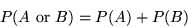Now suppose I throw five coins in the air. What's the probability that at least one of them will show heads? To calculate this probability, we could use the addition rule over and over again, but this gets rather complicated. A much simpler approach is to calculate the probability of this event not happening--that is, the probability that none of the coins will show heads. Since the probability of a single coin showing tails is 1/2, the probability of all five coins showing tails is 1/2 x 1/2 x 1/2 x 1/2 x 1/2 = 1/32. The outcomes in which at least one coin shows heads include all the possible outcomes except the one in which I get five tails. So since the probabilities for all the possible outcomes must add up to 1, the probability that at least one coin shows heads is 1 minus the probability of getting five tails (1 - 1/32 = 31/32).

In general, the probability of an event not occurring is 1 minus the probability of the event occurring. We can express this as a subtraction rule: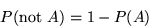1. Consider an ordinary pack of 52 playing cards. You draw a card at random from the pack. What is the probability that it is a queen?
2. What is the probability that it is either a queen or a black ace?
3. What is the probability that it is either a queen or a heart?
4. What is the probability that it is neither a queen nor a heart?

## §5. Conditional probability

Suppose I pick a card at random from a pack of playing cards, without showing you. I ask you to guess which card it is, and you guess the five of diamonds. What is the probability that you are right? Since there are 52 cards in a pack, and only one five of diamonds, the probability of the card being the five of diamonds is 1/52. Next, I tell you that the card is red, not black. Now what is the probability that you are right? Clearly you now have a better chance of being right than you had before. In fact, your chance of being right is twice as big as it was before, since only half of the 52 cards are red. So the probability of the card being the five of diamonds is now 1/26. What we have just calculated is a conditional probability--the probability that the card is the five of diamonds, given that it is red.

If we let A stand for the card being the five of diamonds, and B stand for the card being red, then the conditional probability that the card is the five of diamonds given that it is red is written P(A|B). The definition of conditional probability is: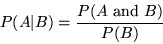In our case, P(A and B) is the probability that the card is the five of diamonds and red, which is 1/52 (exactly the same as P(A), since there are no black fives of diamonds!). P(B), the probability that the card is red, is 1/2. So the definition of conditional probability tells us that P(A|B) = 1/26, exactly as it should. In this simple case we didn't really need to use a formula to tell us this, but the formula is very useful in more complex cases.

If we rearrange the definition of conditional probability, we obtain the multiplication rule for probabilities: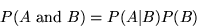One might have expected that the probability of A and B would be obtained by simply multiplying the probabilities of A and B, but in fact this only works in special cases. For example, suppose A stands for "the person speaks Cantonese" and B stands for "the person is from Hong Kong". Suppose we pick a person at random from the world's population, and ask what the value of P(A and B) is. The probability that the person speaks Cantonese is small--the proportion of Cantonese speakers in the world is about 0.01. The probability that the person is from Hong Kong is even smaller--about 0.001. If we multiplied these probabilities together, we would get 0.00001, but this is clearly the wrong way to calculate the probability that the person is both from Hong Kong and a Cantonese speaker.

If we use the definition of conditional probability, we can see the mistake. P(A|B) is the conditional probability that a person speaks Cantonese given that they're from Hong Kong. This number is close to 1. So the correct estimate of the value of P(A and B) is about the same as P(B), the probability that the person is from Hong Kong.

If instead A stands for "the person is female" and B stands for "the person was born in March" then the situation changes. The probability that a person picked at random is female is roughly 1/2, and the probability that the person was born in March is roughly 1/12. The probability P(A and B) that the person is both female and born in March is about 1/24, since about half the people born in March are female. In this case, the probability of A and B is obtained by multiplying the probabilities of A and B. The difference between this case and the last one is that a person's sex and birth date are independent (as far as I know!), whereas a person's native language and where they come from are clearly not independent.

In terms of the multiplication rule, if A and B are independent, then the conditional probability P(A|B) is the same as P(A). (The probability that a person is female given that they were born in March is just the same as the probability that the person is female.) So for independent events, we have a special multiplication rule:
We (implicitly) used the special multiplication rule earlier on, when we calculated that the probability that five tossed coins all show tails is 1/2 x 1/2 x 1/2 x 1/2 x 1/2 = 1/32. In doing so, we assumed that the results of the five tosses are all independent of each other.

1. Suppose you throw two dice, one after the other. What is the probability that the first die shows a 2?
2. What is the probability that the second dice shows a 2?
3. What is the probability that both dice show a 2?
4. What is the probability that the dice add up to 4?
5. What is the probability that the dice add up to 4 given that the first die shows a 2?
6. What is the probability that the dice add up to 4 and the first die shows a 2?